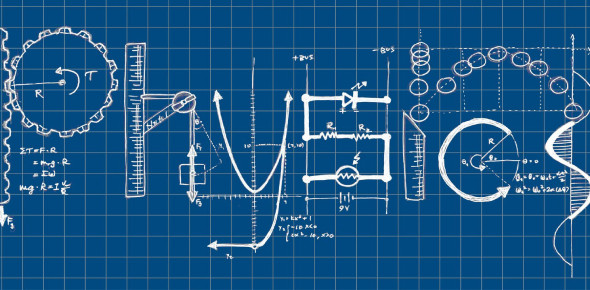# Quiz: Basic Physics Practice Questions!

20 Questions | Attempts: 3815
ShareSettingsAre you interested in fundamental physics? Would you like to try this quiz? This quiz involves you knowing what the atomic number of an element is determined by, what is an ion, what is an isotope, what is a molecule, what is true about a compound, can an electrical insulator be made a conductor, and what are the worst and best conductors. This quiz will teach you basic physics. Good luck.

• 1.
The atomic number of an element is determined by
• A.

The number of neutrons.

• B.

The number of protons.

• C.

The number of neutrons plus the number of protons.

• D.

The number of electrons.

• 2.
The atomic weight of an element is approximately determined by
• A.

The number of neutrons.

• B.

The number of protons.

• C.

The number of neutrons plus the number of protons.

• D.

The number of electrons.

• 3.
Suppose there is an atom of oxygen, containing eight protons and eight neutrons in thenucleus, and two neutrons are added to the nucleus. What is the resulting atomic weight?
• A.

8

• B.

10

• C.

16

• D.

18

• 4.
An ion
• A.

Is electrically neutral.

• B.

Has positive electric charge.

• C.

Has negative electric charge.

• D.

Can have either a positive or negative charge.

• 5.
An isotope
• A.

Is electrically neutral.

• B.

Has positive electric charge.

• C.

Has negative electric charge.

• D.

Can have either a positive or negative charge.

• 6.
A molecule
• A.

Can consist of a single atom of an element.

• B.

Always contains two or more elements.

• C.

Always has two or more atoms.

• D.

Is always electrically charged.

• 7.
In a compound,
• A.

There can be a single atom of an element.

• B.

There must always be two or more elements.

• C.

The atoms are mixed in with each other but not joined.

• D.

There is always a shortage of electrons.

• 8.
An electrical insulator can be made a conductor
• A.

By heating it.

• B.

By cooling it.

• C.

By ionizing it.

• D.

By oxidizing it.

• 9.
Of the following substances, the worst conductor is
• A.

Air.

• B.

Copper.

• C.

Iron.

• D.

Salt water.

• 10.
Of the following substances, the best conductor is
• A.

Air.

• B.

Copper.

• C.

Iron.

• D.

Salt water.

• 11.
Movement of holes in a semiconductor
• A.

Is like a flow of electrons in the same direction.

• B.

Is possible only if the current is high enough.

• C.

Results in a certain amount of electric current.

• D.

Causes the material to stop conducting.

• 12.
If a material has low resistance, then
• A.

It is a good conductor.

• B.

It is a poor conductor.

• C.

The current flows mainly in the form of holes.

• D.

Current can flow only in one direction.

• 13.
A coulomb
• A.

Represents a current of 1 ampere.

• B.

Flows through a 100-watt light bulb.

• C.

Is equivalent to 1 ampere per second.

• D.

Is an extremely large number of charge carriers.

• 14.
A stroke of lightning
• A.

Is caused by a movement of holes in an insulator.

• B.

Has a very low current.

• C.

Is a discharge of static electricity.

• D.

Builds up between clouds.

• 15.
The volt is the standard unit of
• A.

Current.

• B.

Charge.

• C.

Electromotive force.

• D.

Resistance.

• 16.
If an EMF of 1 volt is placed across a resistance of 2 ohms, then the current is
• A.

Half an ampere.

• B.

1 ampere.

• C.

2 amperes.

• D.

Impossible to determine.

• 17.
A backward-working electric motor, in which mechanical rotation is converted to electricity,is best described as
• A.

An inefficient, energy-wasting device.

• B.

A motor with the voltage connected the wrong way.

• C.

An electric generator.

• D.

A magnetic field.

• 18.
In a battery, chemical energy can sometimes be replenished by
• A.

Connecting it to a light bulb.

• B.

Charging it.

• C.

Discharging it.

• D.

No means known; when a battery is dead, you must throw it away.

• 19.
A fluctuating magnetic field
• A.

Produces an electric current in an insulator.

• B.

Magnetizes the earth.

• C.

Produces a fluctuating electric field.

• D.

Results from a steady electric current.

• 20.
Visible light is converted into electricity
• A.

In a dry cell.

• B.

In a wet cell.

• C.

In an incandescent bulb.

• D.

In a photovoltaic cell.

## Related TopicsBack to top
×

Wait!
Here's an interesting quiz for you.